# On the Data-Efficiency with Contrastive Image Transformation in Reinforcement Learning

Sicong Liu1 2 3 *, Xi Sheryl Zhang2 3 5†, Yushuo Li2, Yifan Zhang2 3 5, Jian Cheng2 3 4,
1NJUST, 2 Institute of Automation, Chinese Academy of Sciences, 3AIRIA, 4School of Future Technology, University of Chinese Academy of Sciences, 5University of Chinese Academy of Sciences, Nanjing ,
*Work done during CASIA internship Corresponding Author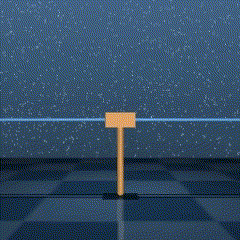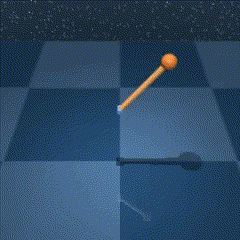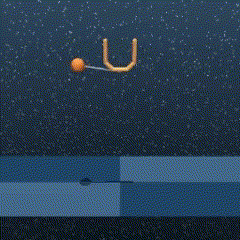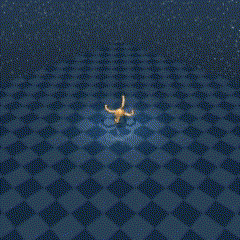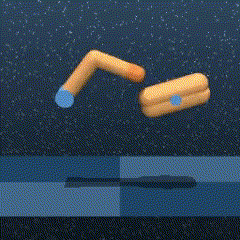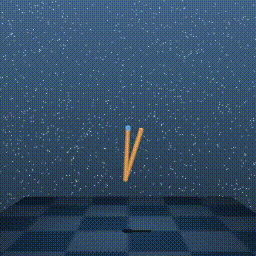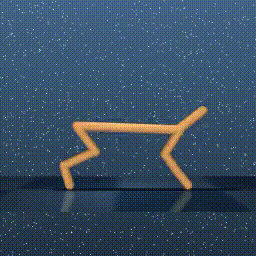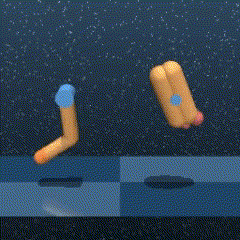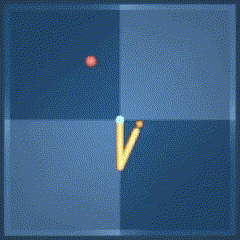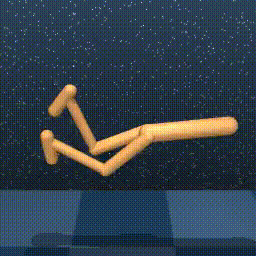## Abstract

Data-efficiency has always been an essential issue in pixel-based reinforcement learning (RL). As the agent not only learns decision-making but also meaningful representations from images. The line of reinforcement learning with data augmentation shows significant improvements in sample-efficiency. However, it is challenging to guarantee the optimality invariant transformation, that is, the augmented data are readily recognized as a completely different state by the agent. In the end, we propose a contrastive invariant transformation (CoIT), a simple yet promising learnable data augmentation combined with standard model-free algorithms to improve sample-efficiency. Concretely, the differentiable CoIT leverages original samples with augmented samples and hastens the state encoder for a contrastive invariant embedding. We evaluate our approach on DeepMind Control Suite and Atari100K. Empirical results verify advances using CoIT, enabling it to outperform the new state-of-the-art on various tasks. Source code is available at https://github.com/mooricAnna/CoIT.

## Method

### Learnable Invariant Transformation

Our method, CoIT introduces a learnable image augmentation to achieve an invariant image transformation. We propose a theoretical analysis of how a learnable invariant image transformation can approximate a stationary distribution over the transformed data by the optimal invariant metric, thus learning better representations.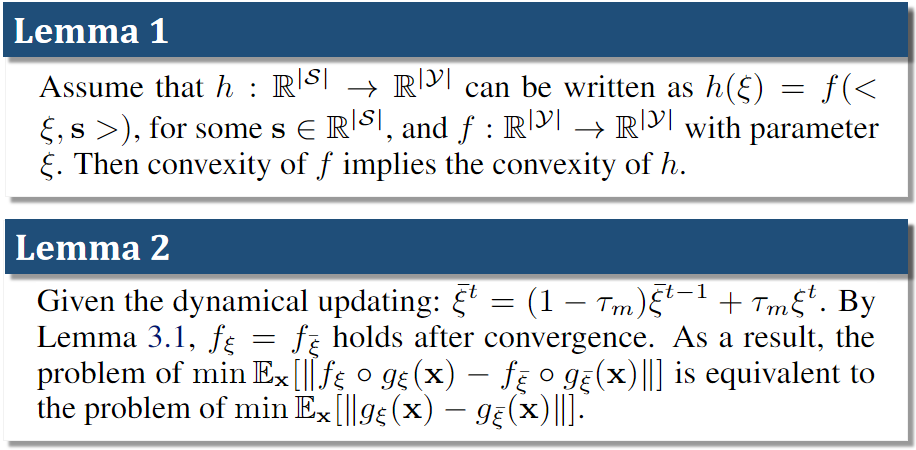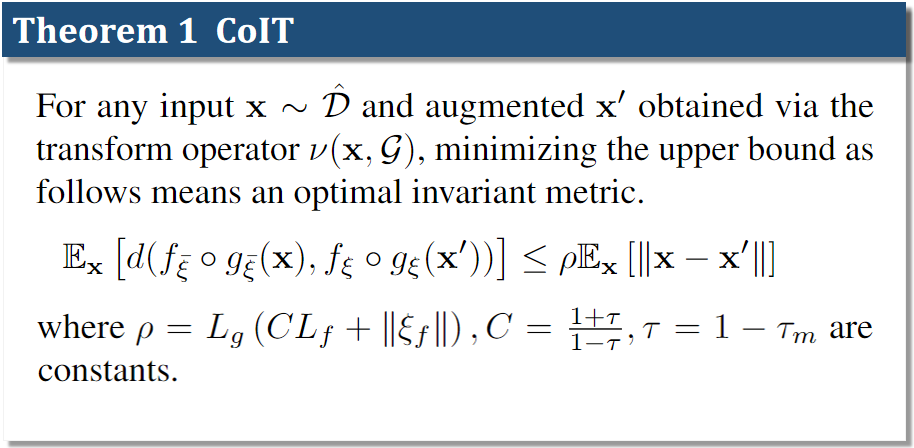### Stabilizing Reward Function

To further stabilize the reward function, we propose a mixed CoIT that samples multiple transformed data from the learned distribution, and mix up them together for model training.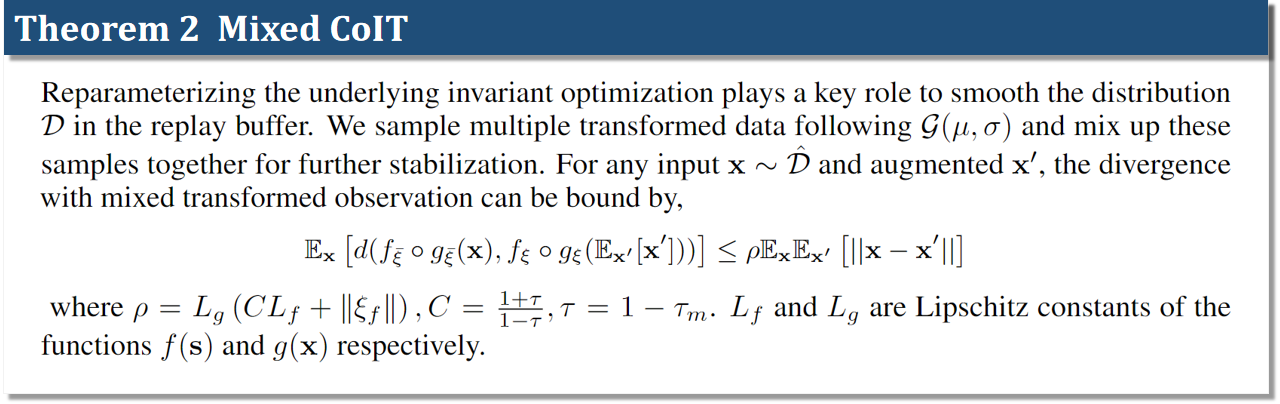### Framework Design

We present a new framework with normalization variants to ensure above discussed learning guarantees by optimizing paraneters. Below are key points of our method.

• We borrow a similar idea from Yin et al. to regularize and smooth the distribution shift between the transformed and overall data. We use the statistical data stored in the BN layers to approximate the distribution of the overall data,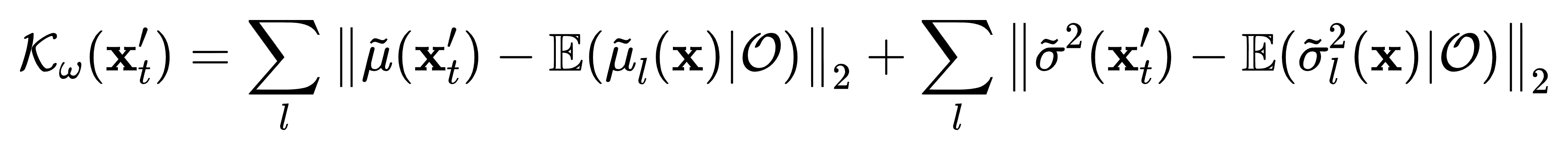where $$\tilde{\mu}(\mathbf{x}_t')$$ and $$\tilde{\sigma}^2(\mathbf{x}_t')$$ are the mean and variance of the transformed data and $$\omega$$ represents the parameters of the learnable image transformation. The expectation terms $$\mathbb{E}(\cdot)$$ denotes the statistical estimation of the batch-wise data stored in the $$l$$-th conv layer, and $$\mathcal{O}$$ is the given observations.
• We utilize the similarity metric proposed by Chen et al. for learning the encoder that encodes the observations into latent space to meet the invariant transformation,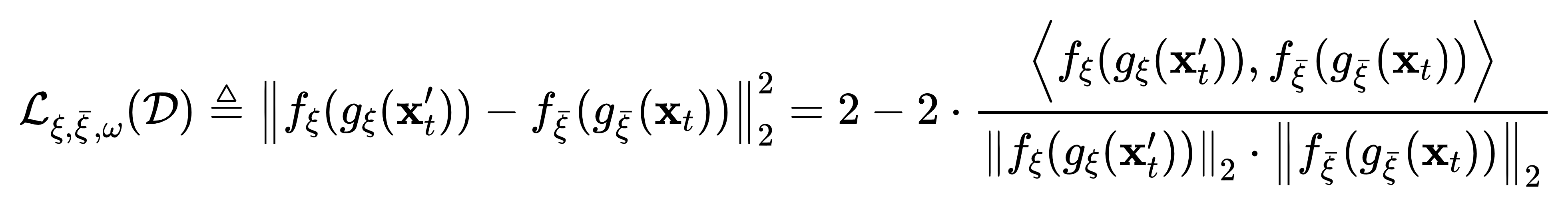Here $$\bar{\xi}$$ denotes the momentum version of parameters $$\xi$$, and $$\mathcal{D}$$ indicates the replay buffer.
• We update the critic network with transformed data $$\mathbf{x}_t'$$ and $$\mathbf{x}_{t+n}'$$ to minimize the TD error for n-steps returns,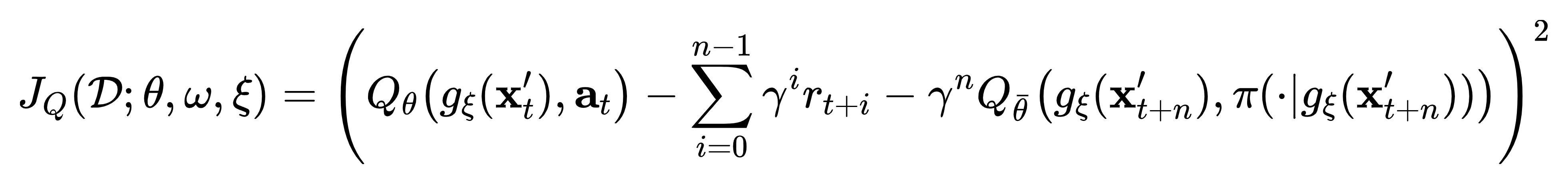Eventually, we give the unified objective function of the CoIT, where $$\alpha$$ and $$\lambda$$ are hyper-parameters and the overall architecture is presented below.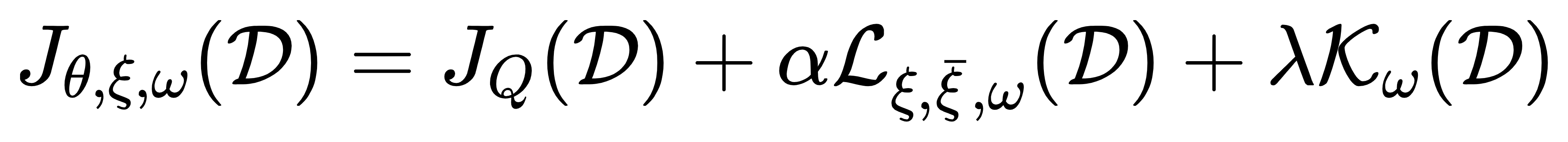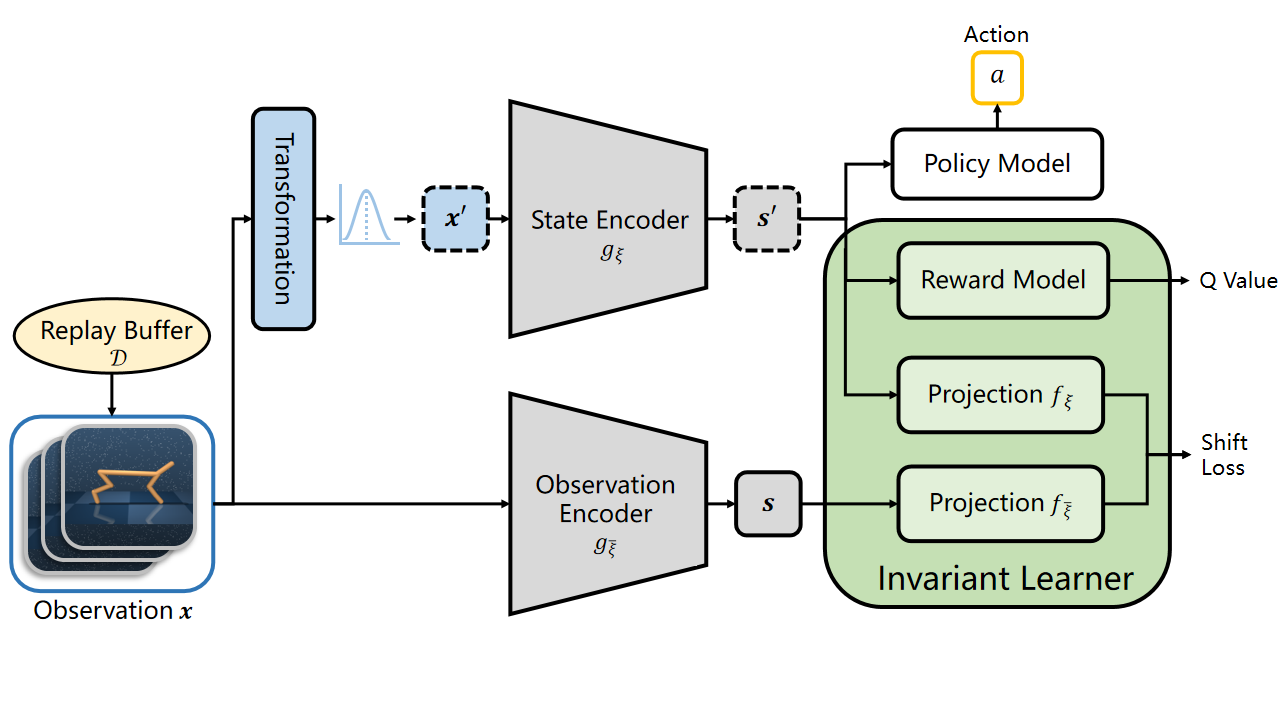Overall architecture of CoIT. The observations are transformed following a Gaussian distribution $$\mathcal{G}(\mu, \sigma)$$ and encoded by the state encoder $$g_\xi$$. The observation encoder $$g_{\bar{\xi}}$$ and projection $$f_{\bar{\xi}}$$ are the exponentially moving average version of the state encoder and projection.

## Experiments

We benchmark our method on the DeepMind control suite and compare CoIT with prior model-free methods which use data augmentation and contrastive learning to improve data-efficiency:

• DrQ-v2: introducing a simple data augmentation called random shift into a fine-tuned DDPG.
• CURL: combining SAC with a self-supervised learning framework.
• Vanilla SAC and DDPG that directly trained from the image input

We also present ablation studies to show the details of our method.

### Evaluation Results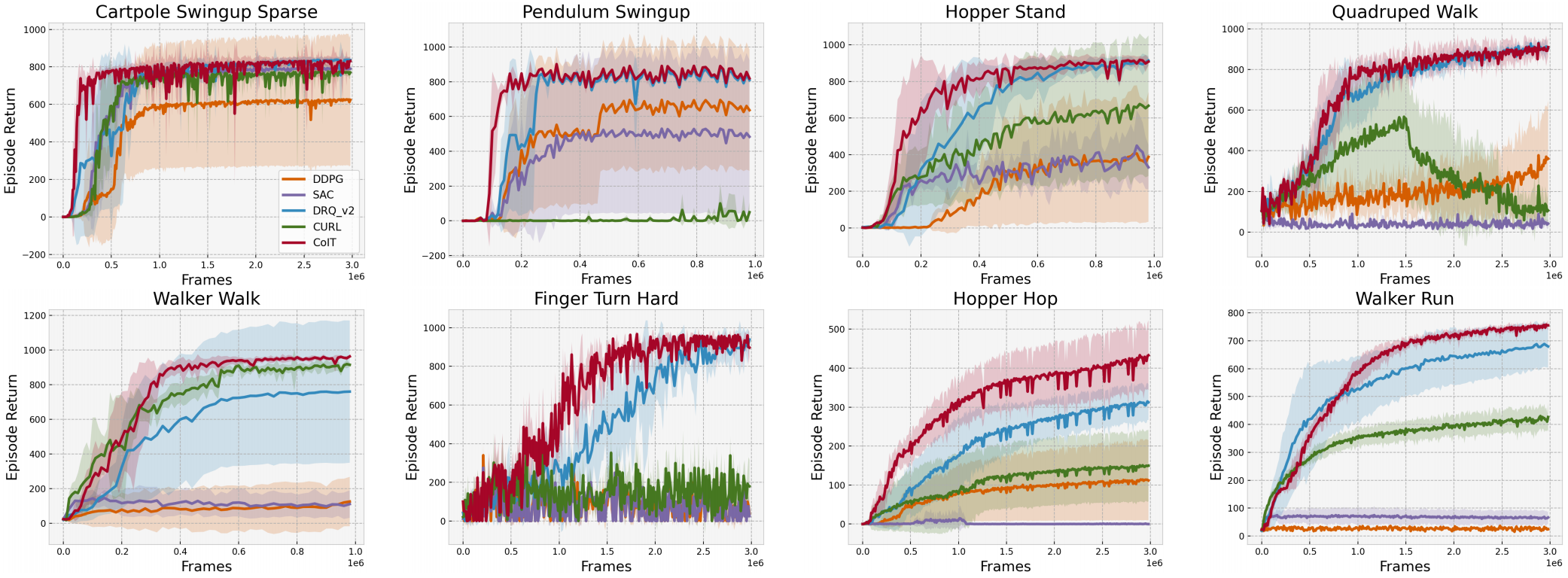Results of 8 complex tasks in DMControl. These tasks are chosen to offer multiple degrees of challenges, including complex dynamics, sparse rewards, hard exploration, and more. Below are key findings: (i) Although DrQ-v2 has already performed remarkably for continuous control, CoIT is more data-efficient on multiple tasks. (ii) From general trends of the learning curves, CoIT improves or keeps the data-efficiency in a more stable manner which is not trivial on DMControl tasks.

### Ablation Studies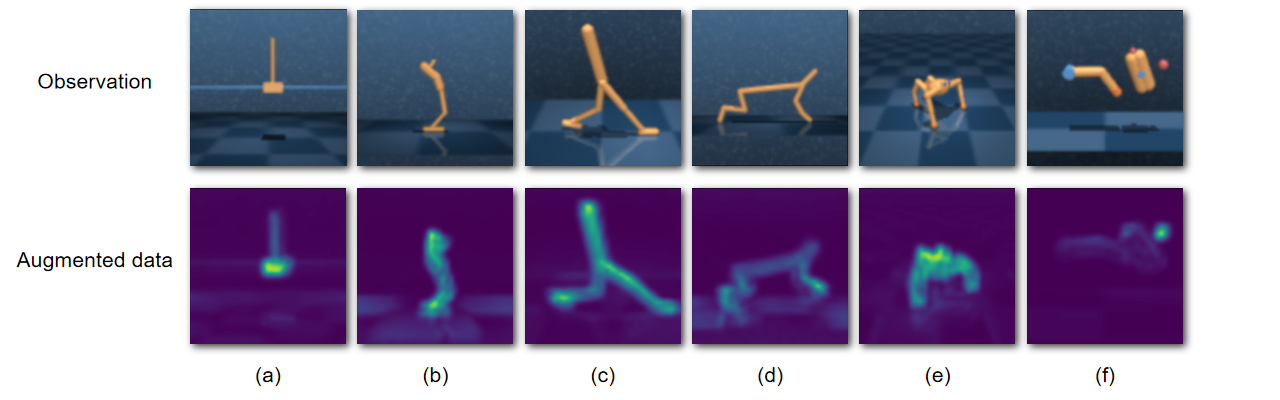Saliency maps for CoIT on 6 tasks: (a) Cartpole Swingup Sparse, (b) Hopper Stand, (c) Walker Run, (d) Cheetah Run, (e) Quadruped Walk, and (f) Finger Turn Hard. These saliency maps demonstrate that CoIT is beneficial for the agent to focus on task-relevant elements like the whole robot body and ignore the task-irrelevant information like the floor and background. Especially in Finger Turn Hard, the lightest part in the saliency map is a red ball in the observation, which is highly related to the reward.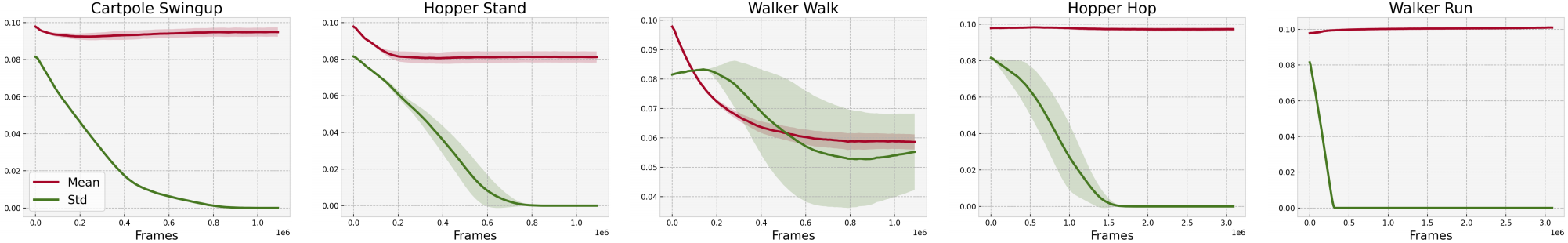Visualization of the parameters of the Gaussian distribution for image transformation. These curves demonstrate that the gaussian distribution proposed in CoIT could automatically find an appropriate transformation to smooth the distribution shift between the different views of the same observation, therefore being beneficial to the representation learning.

## Conclusion

A novel pixel transformation CoIT under model-free RL algorithms that significantly improves the data-efficiency and stability for visual tasks is introduced in this work. We theoretically analyze how the learnable transformation constrains the distribution of the abstracted data, and dissect its benefits to representation learning. CoIT is no need for any additional modifications to the backbone RL algorithm and is easy to implement. We compare CoIT to SOTA methods on popular benchmarks and certify that it gains promising performance with advanced stability. Hopefully, contrastive invariant transformation can lead to a new branch for representation learning in RL.

## BibTeX

            
@inproceedings{liudata,
title={On the Data-Efficiency with Contrastive Image Transformation in Reinforcement Learning},
author={Liu, Sicong and Zhang, Xi Sheryl and Li, Yushuo and Zhang, Yifan and Cheng, Jian},
booktitle={The Eleventh International Conference on Learning Representations}
}